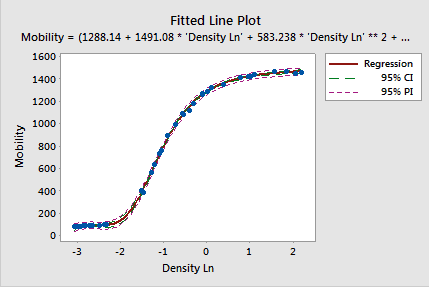Nonlinear regression is a very powerful analysis that can fit virtually any curve. However, it's not possible to calculate a valid R-squared for nonlinear regression. This topic gets complicated because, while Minitab statistical software doesn’t calculate R-squared for nonlinear regression, some other packages do.

So, what’s going on?

Minitab doesn't calculate R-squared for nonlinear models because the research literature shows that it is an invalid goodness-of-fit statistic for this type of model. There are bad consequences if you use it in this context.

## Why Is It Impossible to Calculate a Valid R-squared for Nonlinear Regression?

R-squared is based on the underlying assumption that you are fitting a linear model. If you aren’t fitting a linear model, you shouldn’t use it. The reason why is actually very easy to understand.

For linear models, the sums of the squared errors always add up in a specific manner: SS Regression + SS Error = SS Total.

This seems quite logical. The variance that the regression model accounts for plus the error variance adds up to equal the total variance. Further, R-squared equals SS Regression / SS Total, which mathematically must produce a value between 0 and 100%.

In nonlinear regression, SS Regression + SS Error do not equal SS Total! This completely invalidates R-squared for nonlinear models, and it no longer has to be between 0 and 100%.

What is the difference between linear and nonlinear regression equations?

## Why Shouldn't You Use R-squared to Evaluate the Fit of Nonlinear Models?

As you can see, the underlying assumptions for R-squared aren’t true for nonlinear regression. Yet, most statistical software packages still calculate R-squared for nonlinear regression. Calculating this statistic in this context is a dubious practice that produces bad outcomes.

Spiess and Neumeyer* performed thousands of simulations for their study that show how using R-squared to evaluate the fit of nonlinear models leads you to incorrect conclusions. You don't want this!

That's why Minitab doesn't offer R-squared for nonlinear regression.

Specifically, this study found the following about using R-squared with nonlinear regression:

• R-squared tends to be uniformly high for both very bad and very good models.
• R-squared and adjusted R-squared do not always increase for better nonlinear models.
• Using R-squared and adjusted R-squared to choose the final model led to the correct model only 28-43% of the time.

Clearly, using R-squared to evaluate and choose a nonlinear model is a bad idea. Additionally, the authors lament the persistence of this practice in some fields of study:

In the field of biochemical and pharmacological literature there is a reasonably high occurrence in the use of R 2 as the basis of arguing against or in favor of a certain model. . . . Additionally, almost all of the commercially available statistical software packages calculate R 2 values for nonlinear fits, which is bound to unintentionally corroborate its frequent use. . . . As a result from this work, we would like to advocate that R 2 should not be reported or demanded in pharmacological and biochemical literature when discussing nonlinear data analysis.

If you're already using Minitab, great. However, if you use statistical software that calculates R-squared for nonlinear regression, don’t trust that statistic!

Instead, compare the standard error of the regression (S), and go with the smaller values.

Learn why there are no P values for the variables in nonlinear regression!

________________________________

Spiess, Andrej-Nikolai, Natalie Neumeyer. An evaluation of R2 as an inadequate measure for nonlinear models in pharmacological and biochemical research: a Monte Carlo approach. BMC Pharmacology. 2010; 10: 6.